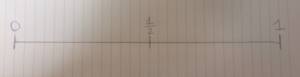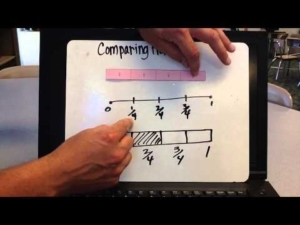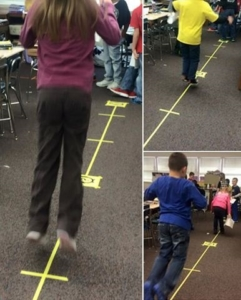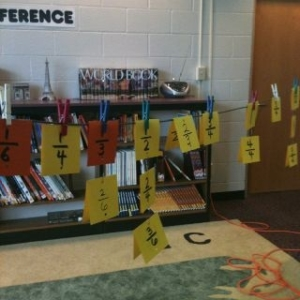Modeling Fractions on a Number Line!

In 3rd grade students are expected to, “Understand a fraction as a number on the number line; represent fractions on a number line.”

This is a concept that students struggle with if you don’t start with concrete models. The best tool to use to help the transition of understanding fractions to modeling fractions on a number line are fraction tiles. Here are the steps to help students develop understanding of fractions on a number line:

Focus on the whole. Have students find the “1 whole” piece so they can relate all other equal parts to the whole.Have students represent different fractions of a whole such as the 3/4 represented below. Spend time describing where its location is as it relates to the whole. For example, “Because my fraction tiles end at this location on the whole, this location represents 3/4.” Continue this process with many different fractions.Have students then trace the whole fraction tile on notebook paper vertically. (Doing this vertically helps create equal intervals on the number line). From there they can determine where the benchmarks 0, 1/2 and 1 whole are located and record that on their paper number line.Students then can choose a fraction to represent on the paper number line. In the example below, students put the 3 tiles that represent 1/4 and record their locations on the paper number line. Since 3/4 is the fraction they are representing, they would put a dot on that location. That dot represents the fraction 3/4.After a lot of exploration and students are demonstrating understanding of how fractions can be represented on a number line, you will want to move them to drawing number lines without the use of the fraction tiles. Support for this can be scaffolded by giving them blank paper number lines or paper number lines with the tick marks already on the number line.Other strategies to use to develop understanding of fractions on a number line:
Walk-on Fraction Number Line. Create a walk-on number line and pose story problems that deal with distance. For example, “Tammy’s house is 1 mile from her school. She has traveled 2/3 of the mile. Where is Tammy at now?” Have students walk on to model the actions in the problem. (Special note – use the floor square tiles to make sure the intervals are equal).String Fraction Number Line. Pass out fraction number cards, string and paper clips. Have students work together to determine the location of each of the fractions on the string number line.Folding Fraction Number Lines. Use sentence strips and have students determine where the 0 and 1 whole are located and record. Then fold the sentence strip into equal parts to determine where 1/2 is located. Fold the sentence strip again down the middle to create fourths. Determine where the different fourths are located, etc. This is best done with the denominators of 2, 4, and 8.For more information on representing fractions on a number line, visit https://learnzillion.com/lesson_plans/6303-identify-a-fraction-as-a-point-on-a-number-line-by-dividing-the-number-line-into-equal-parts or https://learnzillion.com/lesson_plans/6248-plot-a-unit-fraction-on-a-number-line.